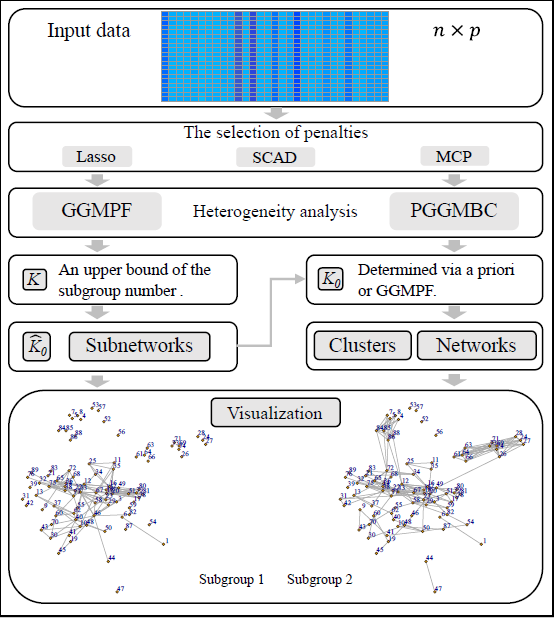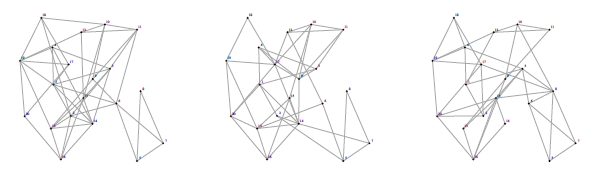# Description

The Gaussian Graphical Model-based (GGM) framework, focusing on the precision matrix and conditional dependence, is a more popular paradigm of heterogeneity analysis, which is more informative than that limited to simple distributional properties. In GGM-based analyses, to determine the number of subgroups is a challenging and important task. This package contains a recently developed and novel method via penalized fusion which can determine the number of subgroups and recover the subgrouping structure fully data-dependently. Moreover, the package also includes some Gaussian graphical mixture model methods requiring a given number of subgroups. The main functions contained in the package are as follows.

• GMMPF: This function implements the GGM-based heterogeneity analysis via penalized fusion (Ren et al., 2021).
• PGGMBC: This method implements the penalized GGM-based clustering with unconstrained covariance matrices (Zhou et al., 2009).
• summary-network: This function provides the summary of the characteristics of the resulting network structures, including the overlap of edges of different subgroups, the connection of node, and so on.
• plot-network: This function implements the visualization of network structures.

We note that the penalties $$p(\cdot, \lambda)$$ used in Ren et al. (2021) and Zhou et al. (2009) are MCP and lasso, respectively. Our package provides the variety of types of penalties for both two methods, including convex and concave penalties. The workflow of the GMMPF package is as follows.# GMMPF

A relatively large number $$K$$, an upper bound of the true number of subgroups $$K_0$$, needs to be set by the users, which is easy to specify based on some biological knowledge. A new fusion penalty is developed to shrink differences of parameters among the $$K$$ subgroups and encourage equality, and then a smaller number of subgroups can be yielded. Three tuning parameters $$\lambda_1$$, $$\lambda_2$$, and $$\lambda_3$$ are involved, where $$\lambda_1$$ and $$\lambda_2$$ are routine to determine the sparsity of parameters in means and precision matrices and regularize estimation. And the conditional dependence relationships for each subgroup can be obtained by examining the nonzero estimates of the resulting precision matrices. $$\lambda_3$$ is a pivotal parameter to control the degree of shrinking differences, which implements the effective searching" between 1 and $$K$$ based on the penalized fusion technique.

## Data setting

Denote $$n$$ as the size of independent subjects. Consider sample $$i(=1,\ldots, n)$$, $$p$$-dimensional measurement $$\boldsymbol{x}_i$$ is available. Further assume that the $$n$$ subjects belong to $$K_0$$ subgroups, where the value of $$K_0$$ is unknown. For the $$l$$th subgroup, assume the Gaussian distribution: $\begin{equation}\nonumber f_{l}\left(\boldsymbol{x} ; \boldsymbol{\mu}_{l}^{*}, \mathbf{\Sigma}_{l}^{*}\right)=(2 \pi)^{-p / 2}\left|\boldsymbol{\Sigma}_{l}^{*}\right|^{-1 / 2} \exp \left\{-\frac{1}{2}\left(\boldsymbol{x}-\boldsymbol{\mu}_{l}^{*}\right)^{\top} (\boldsymbol{\Sigma}_{l}^{*})^{-1}\left(\boldsymbol{x}-\boldsymbol{\mu}_{l}^{*}\right)\right\}, \end{equation}$ where the mean and covariance matrix are unknown. Overall, $$\boldsymbol{x}_i$$s satisfy distribution: $\begin{equation}\nonumber f(\boldsymbol{x}) =\sum_{l=1}^{K_0} \pi_{l}^{*} f_{l}\left(\boldsymbol{x} ; \boldsymbol{\mu}_{l}^{*}, \mathbf{\Sigma}_{l}^{*}\right), \end{equation}$ where the mixture probabilities $$\pi_{l}^{*}$$s are also unknown. Our goal is to determine the number of subgroups $$K_0$$ and estimate the subgrouping structure fully data-dependently.

## Method

GGM-based heterogeneity analysis via penalized fusion is based on the penalized objective function: $\begin{equation}\label{obj} \mathcal{L}(\boldsymbol{\Omega}, \boldsymbol{\pi} | \boldsymbol{X} ):= \frac{1}{n} \sum_{i=1}^{n} \log \left(\sum_{k=1}^{K} \pi_{k} f_{k}\left( \boldsymbol{x}_{i} ; \boldsymbol{\mu}_{k},\boldsymbol{\Theta}_{k}^{-1}\right)\right) - \mathcal{P}(\boldsymbol{\Omega}), \end{equation}$ where $$\boldsymbol{X}$$ denotes the collection of observed data, $$\boldsymbol{\Omega} = (\boldsymbol{\Omega}_1^{\top}, \cdots, \boldsymbol{\Omega}_K^{\top} )^{\top}$$, $$\boldsymbol{\Omega}_k=\operatorname{vec}\left(\boldsymbol{\mu}_{k}, \boldsymbol{\Theta}_{k}\right)=\left(\mu_{k 1}, \ldots, \mu_{k p}, \theta_{k 11}, \ldots, \theta_{k p 1}, \ldots, \theta_{k 1 p}, \ldots, \theta_{k p p}\right) \in \mathbb{R}^{p^{2}+p}$$, $$\boldsymbol{\Theta}_{k}=\boldsymbol{\Sigma}_{k}^{-1}$$ is the $$k$$-th precision matrix with the $$ij$$-th entry $$\theta_{kij}$$, $$\boldsymbol{\pi} = (\pi_{1}, \cdots, \pi_{K})^{\top}$$, \begin{equation}\label{penalty} %\begin{aligned} \mathcal{P}(\boldsymbol{\Omega}) = %& \sum_{k=1}^{K} \sum_{j=1}^{p} p(|\mu_{kj}|, \lambda_{1}) + \sum_{k=1}^{K} \sum_{i \neq j} p(\left|\theta_{k i j}\right|, \lambda_{2}) %\\ %& + \sum_{k < k^{\prime}} p \left( \left( \|\boldsymbol{\mu}_{k} - \boldsymbol{\mu}_{k^{\prime}}\|_2^2 + \| \boldsymbol{\Theta}_{k} - \boldsymbol{\Theta}_{k^{\prime}}\|_F^2 \right)^{1/2}, \lambda_{3} \right), %\end{aligned} \end{equation} $$\|\cdot\|_F$$ is the Frobenius norm, and $$p(\cdot, \lambda)$$ is a penalty function with tuning parameter $$\lambda > 0$$, which can be selected as lasso, SCAD, MCP, and others. $$K$$ is a known constant that satisfies $$K>K_0$$. Consider: $\begin{equation}\nonumber (\widehat{\boldsymbol{\Omega}}, \widehat{\boldsymbol{\pi}} )=\underset{ \boldsymbol{\Omega}, \boldsymbol{\pi} }{ \mathrm{argmax}} \mathcal{L}(\boldsymbol{\Omega}, \boldsymbol{\pi} | \boldsymbol{X} ). \end{equation}$ Denote $$\{\widehat{\boldsymbol{\Upsilon}}_1 , \cdots, \widehat{\boldsymbol{\Upsilon}}_{\widehat{K}_0} \}$$ as the distinct values of $$\widehat{\boldsymbol{\Omega}}$$, that is, $$\{k: \widehat{\boldsymbol{\Omega}}_k \equiv \widehat{\boldsymbol{\Upsilon}}_l, k=1, \cdots, K \}_{ l=1, \cdots, \widehat{K}_0 }$$ constitutes a partition of $$\{1, \cdots, K\}$$. Then there are $$\widehat{K}_0$$ subgroups with estimated mean and precision parameters in $$\widehat{\boldsymbol{\Omega}}$$. The mixture probabilities can be extracted from $$\widehat{\boldsymbol{\pi}}$$.

## Example

First, we call the built-in simulation data set ($$K_0 = 3$$), and set the upper bound of $$K_0$$ and the sequences of the tuning parameters ($$\lambda1$$, $$\lambda2$$, and $$\lambda3$$).

data(example.data)
K <- 6
lambda <- genelambda.obo(nlambda1=5,lambda1_max=0.5,lambda1_min=0.1,
nlambda2=15,lambda2_max=1.5,lambda2_min=0.1,
nlambda3=10,lambda3_max=3.5,lambda3_min=0.5)

Apply GGMPF to the data.

set.seed(1)
res <- GGMPF(lambda, example.data$data, K, penalty = "MCP") Theta_hat.list <- res$Theta_hat.list
Mu_hat.list <- res$Mu_hat.list opt_num <- res$Opt_num
opt_Mu_hat <- Mu_hat.list[[opt_num]]
opt_Theta_hat <- Theta_hat.list[[opt_num]]
K_hat <- dim(opt_Theta_hat)
K_hat  # Output the estimated K0.

Summarize the characteristics of the resulting network structures, and implement visualization of network structures.

summ <- summary_network(opt_Mu_hat, opt_Theta_hat, example.data$data) summ$Theta_summary\$overlap
va_names <- c("6")
plot_network(summ, num_subgroup = c(1:K_hat))